Courses

# Two Hinged Arch (Part - 2) Civil Engineering (CE) Notes | EduRev

## Civil Engineering (CE) : Two Hinged Arch (Part - 2) Civil Engineering (CE) Notes | EduRev

The document Two Hinged Arch (Part - 2) Civil Engineering (CE) Notes | EduRev is a part of the Civil Engineering (CE) Course Structural Analysis.
All you need of Civil Engineering (CE) at this link: Civil Engineering (CE)

Temperature effect

Consider an unloaded two-hinged arch of span L . When the arch undergoes a uniform temperature change of T°C , then its span would increase by α L T if it were allowed to expand freely (vide Fig 33.3a). α is the co-efficient of thermal expansion of the arch material. Since the arch is restrained from the horizontal movement, a horizontal force is induced at the support as the temperature is increased.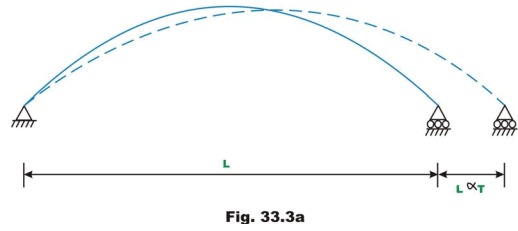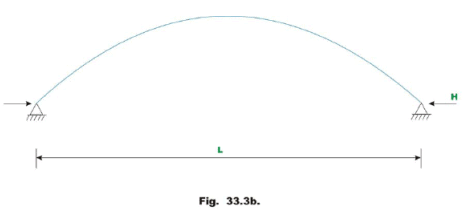Now applying the Castigliano’s first theorem,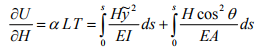(33.12)

Solving for H ,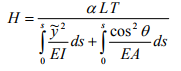(33.13)

The second term in the denominator may be neglected, as the axial rigidity is quite high. Neglecting the axial rigidity, the above equation can be written as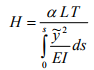(33.14)

Example 33.1
A semicircular two hinged arch of constant cross section is subjected to a concentrated load as shown in Fig 33.4a. Calculate reactions of the arch and draw bending moment diagram.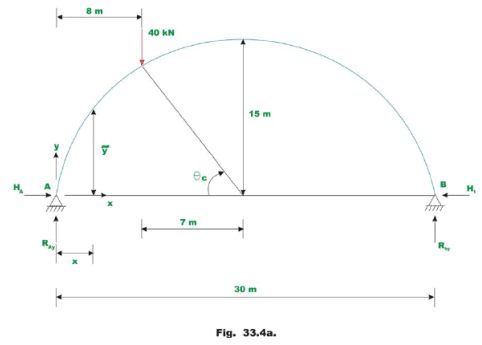Solution: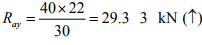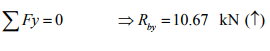(1)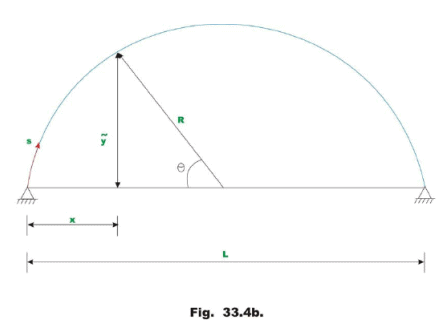From Fig. 33.4b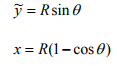ds = R dθ                                                (2)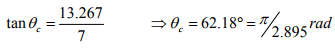Now, the horizontal reaction H may be calculated by the following expression,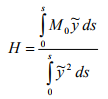(3)

Now M0 the bending moment at any cross section of the arch when one of the hinges is replaced by a roller support is given by,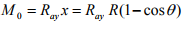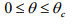and,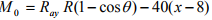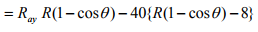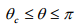(4)

Integrating the numerator in equation (3),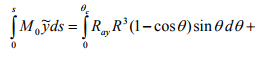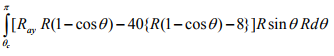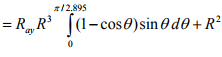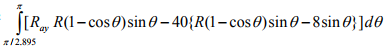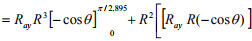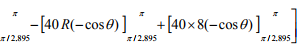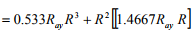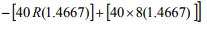= 52761.00 + 225(645.275 − 410.676) = 105545 .775                       (5)

The value of denominator in equation (3), after integration is,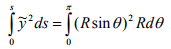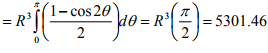(6)

Hence, the horizontal thrust at the support is,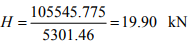(7)

Bending moment diagram

Bending moment M at any cross section of the arch is given by,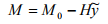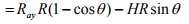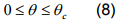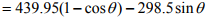M = 439.95(1 − cosθ) − 298.5 sinθ − 40(15(1 − cosθ ) − 8)          θc ≤ θ ≤ π                    (9)

Using equations (8) and (9), bending moment at any angle θ can be computed. The bending moment diagram is shown in Fig. 33.4c.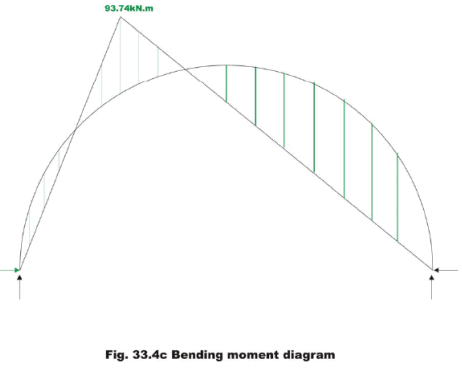Example 33.2
A two hinged parabolic arch of constant cross section has a span of 60m and a rise of 10m. It is subjected to loading as shown in Fig.33.5a. Calculate reactions of the arch if the temperature of the arch is raised by 40°C. Assume co-efficient of thermal expansion as α = 12 × 10-6/ °C.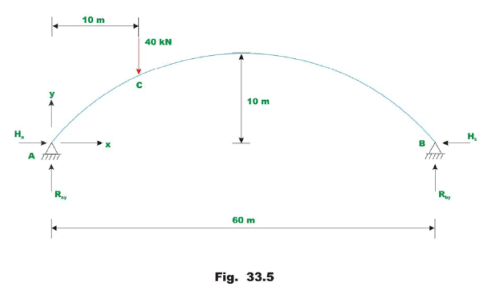Taking A as the origin, the equation of two hinged parabolic arch may be written as,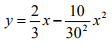(1)

The given problem is solved in two steps. In the first step calculate the horizontal reaction due to 40kN load applied at C. In the next step calculate the horizontal reaction due to rise in temperature. Adding both, one gets the horizontal reaction at the hinges due to combined external loading and temperature change. The horizontal reaction due to 40kN load may be calculated by the following equation,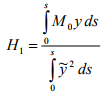(2a)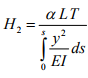(2b)

Where L is the span of the arch.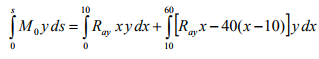(3)

Please note that in the above equation, the integrations are carried out along the x-axis instead of the curved arch axis. The error introduced by this change in the variables in the case of flat arches is negligible. Using equation (1), the above equation (3) can be easily evaluated.

The vertical reaction A is calculated by taking moment of all forces about B . Hence,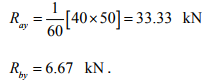Now consider the equation (3),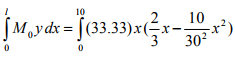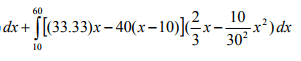= 6480.76 + 69404.99 = 74885.75                                   (4)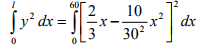(5)

= 3200

Hence, the horizontal reaction due to applied mechanical loads alone is given by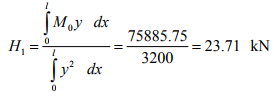(6)

The horizontal reaction due to rise in temperature is calculated by equation (2b),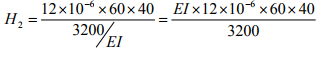Taking E = 200 kN/mm2 and I = 0.0333 m4

H2 = 59.94 kN.                              (7)

Hence the total horizontal thrust H =H1 + H2 = 83.65 kN.

When the arch shape is more complicated, the integrations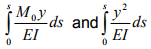are accomplished numerically. For this purpose, divide the arch span in to equals divisions. Length of each division is represented by (Δs) (vide Fig.33.5b). At the midpoint of each division calculate the ordinate  yi by using the equation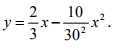The above integrals are approximated as,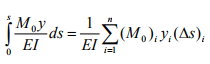(8)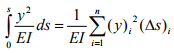(9)

The complete computation for the above problem for the case of external loading is shown in the following table.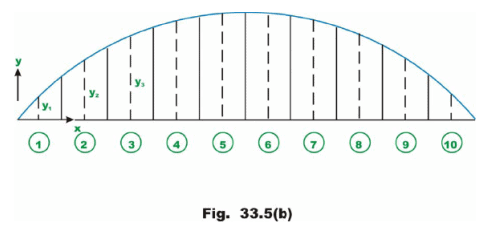Table 1. Numerical integration of equations (8) and (9)

 Segme nt No Horizontal distance x Measured from A (m) Correspond ing yi (m) Moment at that Point (M0)i (kNm) (M0)i yi (Δs)i (y)i2 (Δs)i 1 3 1.9 9 9.99 1139 .886 21.66 2 9 5.1 29 9.97 9179 .082 156.06 3 15 7.5 2 99.95 1349 7.75 337.5 4 21 9.1 2 59.93 1419 2.18 496.86 5 27 9.9 2 19.91 1306 2.65 588.06 6 33 9.9 1 79.89 1068 5.47 588.06 7 39 9.1 1 39.87 7636 .902 496.86 8 45 7.5 9 9.85 449 3.25 337.5 9 51 5.1 5 9.83 183 0.798 156.06 10 57 1.9 19.81 22 5.834 21.66 ∑ 75943.8 3300.3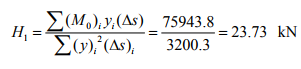(10)

This compares well with the horizontal reaction computed from the exact integration.

Summary
Two-hinged arch is the statically indeterminate structure to degree one. Usually, the horizontal reaction is treated as the redundant and is evaluated by the method of least work. Towards this end, the strain energy stored in the twohinged arch during deformation is given. The reactions developed due to thermal loadings are discussed. Finally, a few numerical examples are solved to illustrate the procedure.

Offer running on EduRev: Apply code STAYHOME200 to get INR 200 off on our premium plan EduRev Infinity!

## Structural Analysis

30 videos|122 docs|28 tests

,

,

,

,

,

,

,

,

,

,

,

,

,

,

,

,

,

,

,

,

,

;